# Descriptive Epidemiology using epiR

#### 2022-09-26

Epidemiology is the study of the frequency, distribution and determinants of health-related states in populations and the application of such knowledge to control health problems .

This vignette provides instruction on the way R and epiR can be used for descriptive epidemiological analyses, that is, to describe how the frequency of disease varies by individual, place and time.

## Indivdual

Descriptions of disease frequency involves reporting either the prevalence or incidence of disease.

Some definitions. Strictly speaking, ‘prevalence’ equals the number of cases of a given disease or attribute that exists in a population at a specified point in time. Prevalence risk is the proportion of a population that has a specific disease or attribute at a specified point in time. Many authors use the term ‘prevalence’ when they really mean prevalence risk, and these notes will follow this convention.

Two types of prevalence are reported in the literature: (1) point prevalence equals the proportion of a population in a diseased state at a single point in time, (2) period prevalence equals the proportion of a population with a given disease or condition over a specific period of time (i.e. the number of existing cases at the start of a follow-up period plus the number of incident cases that occur during the follow-up period).

Incidence provides a measure of how frequently susceptible individuals become disease cases as they are observed over time. An incident case occurs when an individual changes from being susceptible to being diseased. The count of incident cases is the number of such events that occur in a population during a defined follow-up period. There are two ways to express incidence:

Incidence risk (also known as cumulative incidence) is the proportion of initially susceptible individuals in a population who become new cases during a defined follow-up period.

Incidence rate (also known as incidence density) is the number of new cases of disease that occur per unit of individual time at risk during a defined follow-up period.

In addition to reporting the point estimate of disease frequency, it is important to provide an indication of the uncertainty around that point estimate. The epi.conf function in the epiR package allows you to calculate confidence intervals for prevalence, incidence risk and incidence rates.

Let’s say we’re interested in the prevalence of disease X in a population comprised of 1000 individuals. Two hundred are tested and four returned a positive result. Assuming 100% test sensitivity and specificity, what is the estimated prevalence of disease X in this population?

library(epiR); library(ggplot2); library(scales); library(zoo)

ncas <- 4; npop <- 200
tmp <- as.matrix(cbind(ncas, npop))
epi.conf(tmp, ctype = "prevalence", method = "exact", N = 1000, design = 1,
conf.level = 0.95) * 100
#>   est     lower    upper
#> 1   2 0.5475566 5.041361

The estimated prevalence of disease X in this population is 2.0 (95% confidence interval [CI] 0.55 to 5.0) cases per 100 individuals at risk.

Another example. A study was conducted by Feychting, Osterlund, and Ahlbom (1998) to report the frequency of cancer among the blind. A total of 136 diagnoses of cancer were made from 22,050 person-years at risk. What was the incidence rate of cancer in this population?

ncas <- 136; ntar <- 22050
tmp <- as.matrix(cbind(ncas, ntar))
epi.conf(tmp, ctype = "inc.rate", method = "exact", N = 1000, design = 1,
conf.level = 0.95) * 1000
#>         est    lower    upper
#> ncas 6.1678 5.174806 7.295817

The incidence rate of cancer in this population was 6.2 (95% CI 5.2 to 7.3) cases per 1000 person-years at risk.

Lets say we want to compare the frequency of disease across several populations. An effective way to do this is to use a ranked error bar plot. With a ranked error bar plot the points represent the point estimate of the measure of disease frequency and the error bars indicate the 95% confidence interval around each estimate. The disease frequency estimates are then sorted from lowest to highest.

Generate some data. First we’ll generate a distribution of disease prevalence estimates. Let’s say it has a mode of 0.60 and we’re 80% certain that the prevalence is greater than 0.35. Use the epi.betabuster function to generate parameters that can be used for a beta distribution to satisfy these constraints:

tmp <- epi.betabuster(mode = 0.60, conf = 0.80, greaterthan = TRUE, x = 0.35,
conf.level = 0.95, max.shape1 = 100, step = 0.001)
tmp$shape1; tmp$shape2
#>  2.357
#>  1.904667

Take 100 draws from a beta distribution using the shape1 and shape2 values calculated above and plot them as a frequency histogram:

dprob <- rbeta(n = 25, shape1 = tmp$shape1, shape2 = tmp$shape2)
dat.df <- data.frame(dprob = dprob)

ggplot(data = dat.df, aes(x = dprob)) +
theme_bw() +
geom_histogram(binwidth = 0.01, colour = "gray", fill = "dark blue", size = 0.1) +
scale_x_continuous(limits = c(0,1), name = "Prevalence") +
scale_y_continuous(limits = c(0,10), name = "Number of draws")
#> Warning: Removed 2 rows containing missing values (geom_bar).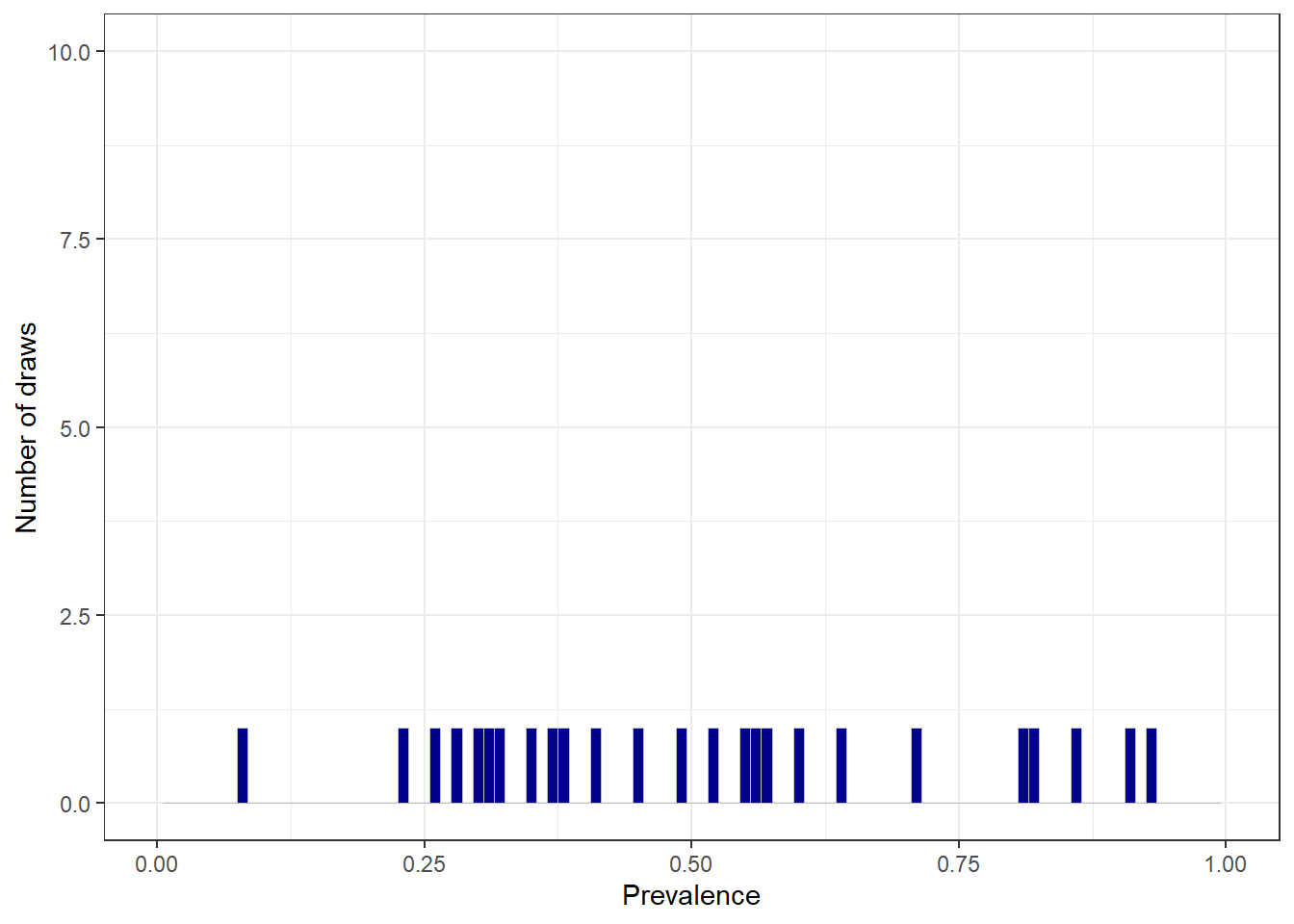Frequency histogram of disease prevalence estimates for our simulated population.

Generate a vector of population sizes using the uniform distribution. Calculate the number of diseased individuals in each population using dprob (calculated above). Finally, calculate the prevalence of disease in each population and its 95% confidence interval using epi.conf. The function epi.conf provides several options for confidence interval calculation methods for prevalence. Here we’ll use the exact method:

dat.df$rname <- paste("Region ", 1:25, sep = "") dat.df$npop <- round(runif(n = 25, min = 20, max = 1500), digits = 0)
dat.df$ncas <- round(dat.df$dprob * dat.df$npop, digits = 0) tmp <- as.matrix(cbind(dat.df$ncas, dat.df$npop)) tmp <- epi.conf(tmp, ctype = "prevalence", method = "exact", N = 1000, design = 1, conf.level = 0.95) * 100 dat.df <- cbind(dat.df, tmp) head(dat.df) #> dprob rname npop ncas est lower upper #> 1 0.6025061 Region 1 239 144 60.25105 53.74182 66.50267 #> 2 0.5640509 Region 2 1065 601 56.43192 53.39267 59.43554 #> 3 0.9297289 Region 3 1201 1117 93.00583 91.41368 94.38315 #> 4 0.5730353 Region 4 760 436 57.36842 53.76376 60.91569 #> 5 0.7083191 Region 5 1083 767 70.82179 68.01425 73.51570 #> 6 0.3466165 Region 6 917 318 34.67830 31.59669 37.85872 Sort the data in order of variable est and assign a 1 to n identifier as variable rank: dat.df <- dat.df[sort.list(dat.df$est),]
dat.df$rank <- 1:nrow(dat.df) Create a ranked error bar plot. Because its useful to provide the region-area names on the horizontal axis we’ll rotate the horizontal axis labels by 90 degrees. ggplot(data = dat.df, aes(x = rank, y = est)) + theme_bw() + geom_errorbar(aes(ymin = lower, ymax = upper), width = 0.1) + geom_point() + scale_x_continuous(limits = c(0,25), breaks = dat.df$rank, labels = dat.df$rname, name = "Region") + scale_y_continuous(limits = c(0,100), name = "Cases per 100 individuals at risk") + theme(axis.text.x = element_text(angle = 90, hjust = 1))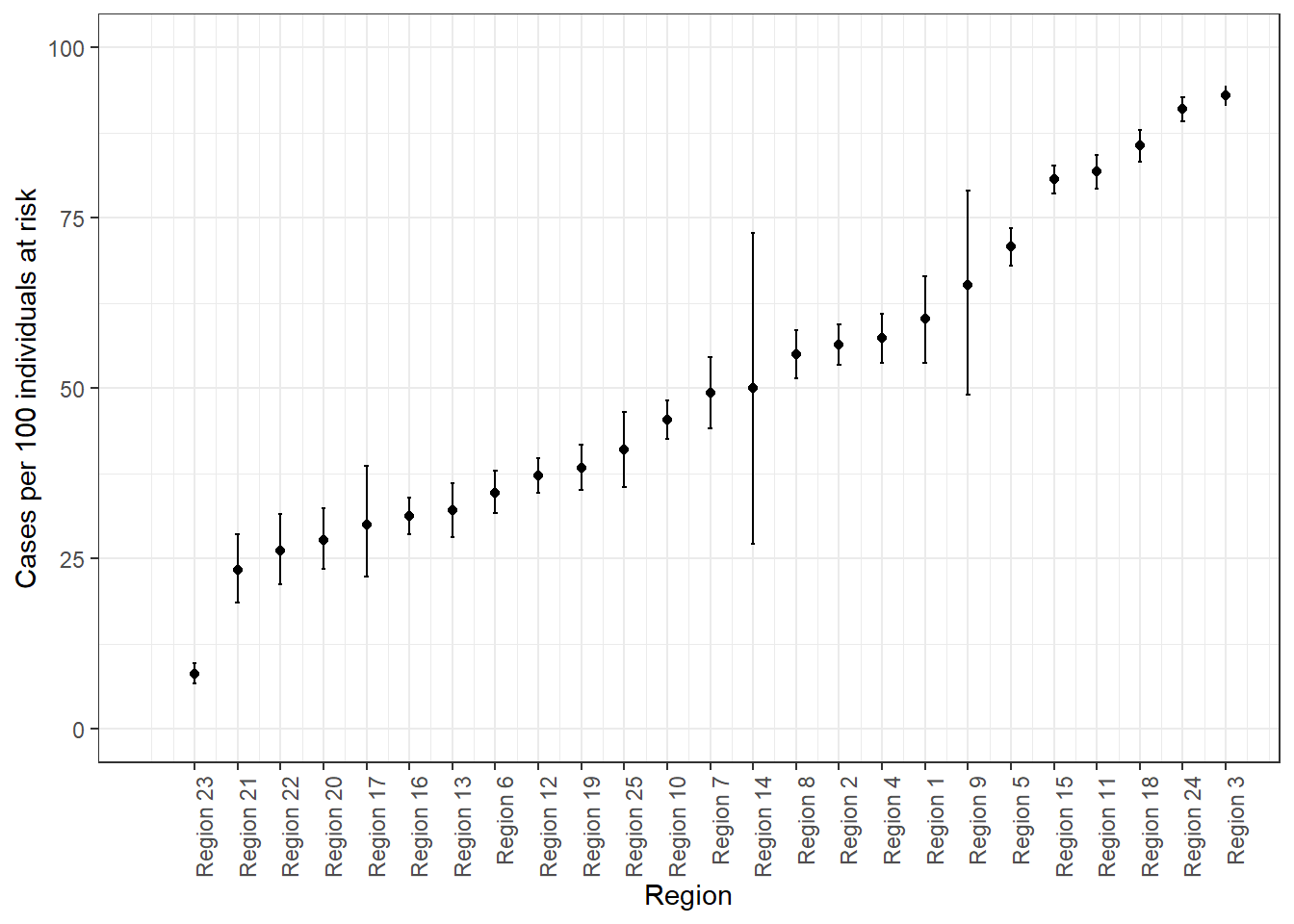Ranked error bar plot showing the prevalence of disease (and its 95% confidence interval) for 100 population units. ## Time Epidemic curves are typically used to describe patterns of disease over time. Epidemic curve data are often presented in one of two formats: 1. One row for each individual identified as a case with an event date assigned to each. 2. One row for every event date with an integer representing the number of cases identified on that date. In the notes that follow we provide details on how to produce an epidemic curve when you’re data are in either of these two formats. ### One row of data for each case Generate some data, with one row for every individual identified as a case: n.males <- 100; n.females <- 50 odate <- seq(from = as.Date("2004-07-26"), to = as.Date("2004-12-13"), by = 1) prob <- c(1:100, 41:1); prob <- prob / sum(prob) modate <- sample(x = odate, size = n.males, replace = TRUE, p = prob) fodate <- sample(x = odate, size = n.females, replace = TRUE) dat.df <- data.frame(sex = c(rep("Male", n.males), rep("Female", n.females)), odate = c(modate, fodate)) # Sort the data in order of odate: dat.df <- dat.df[sort.list(dat.df$odate),] 

Plot the epidemic curve using the ggplot2 and scales packages:

ggplot(data = dat.df, aes(x = as.Date(odate))) +
theme_bw() +
geom_histogram(binwidth = 7, colour = "gray", fill = "dark blue", size = 0.1) +
scale_x_date(breaks = date_breaks("7 days"), labels = date_format("%d %b"),
name = "Date") +
scale_y_continuous(breaks = seq(from = 0, to = 20, by = 2), limits = c(0,20), name = "Number of cases") +
theme(axis.text.x = element_text(angle = 90, hjust = 1))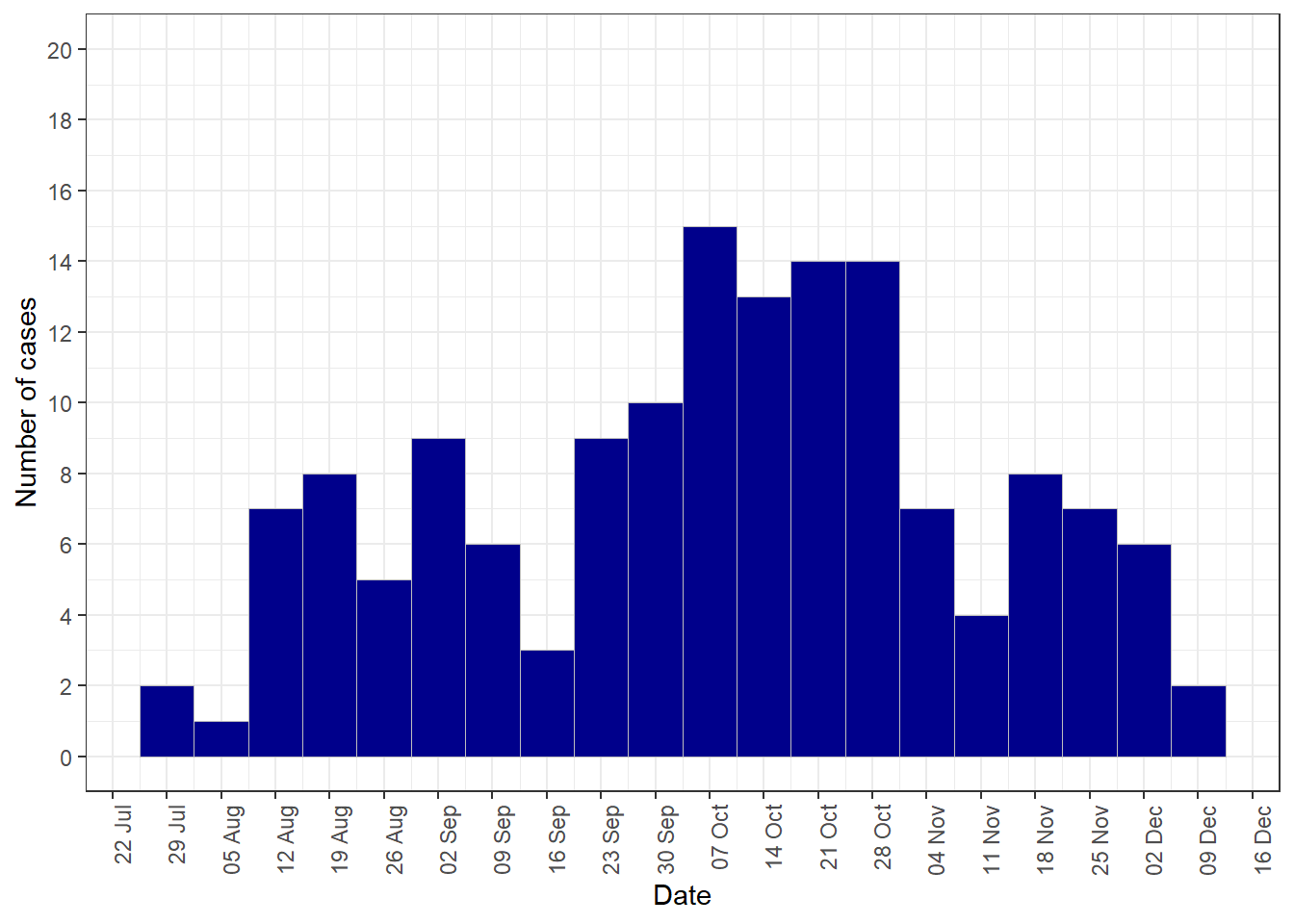Frequency histogram showing counts of incident cases of disease as a function of calendar date, 26 July to 13 December 2004.

You may want to superimpose a smoothed line to better appreciate trend. Do this using the geom_density function in ggplot2:


ggplot(data = dat.df, aes(x = odate)) +
theme_bw() +
geom_histogram(binwidth = 7, colour = "gray", fill = "dark blue", size = 0.1) +
geom_density(aes(y = ..density.. * (nrow(dat.df) * 7)), colour = "red") +
scale_x_date(breaks = date_breaks("7 days"), labels = date_format("%d %b"),
name = "Date") +
scale_y_continuous(breaks = seq(from = 0, to = 20, by = 2), limits = c(0,20), name = "Number of cases") +
theme(axis.text.x = element_text(angle = 90, hjust = 1))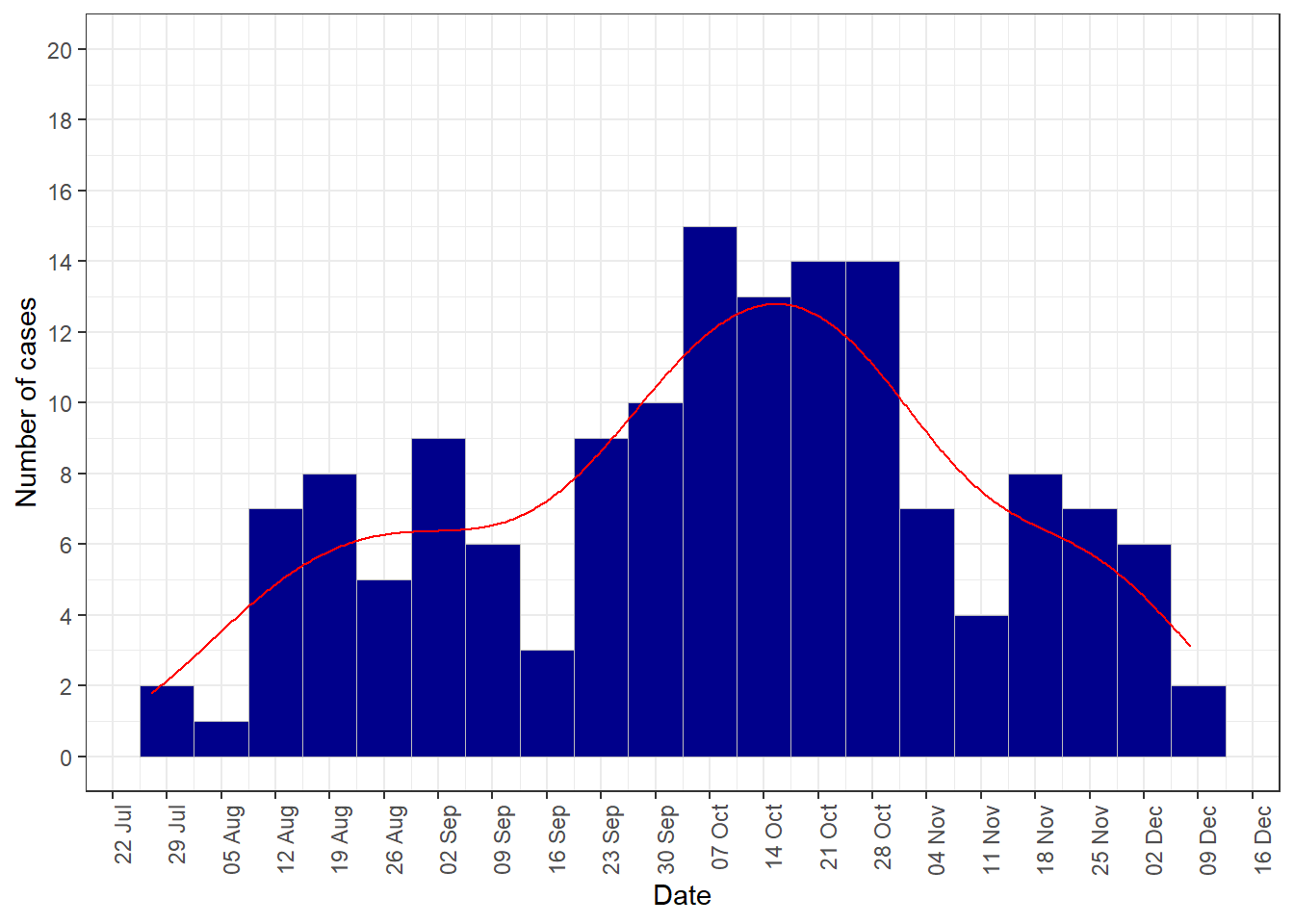Frequency histogram showing counts of incident cases of disease as a function of calendar date, 26 July to 13 December 2004. Superimposed on this plot is a smoothed estimate of case density.

Produce a separate epidemic curve for males and females using the facet_grid option in ggplot2:

ggplot(data = dat.df, aes(x = as.Date(odate))) +
theme_bw() +
geom_histogram(binwidth = 7, colour = "gray", fill = "dark blue", size = 0.1) +
scale_x_date(breaks = date_breaks("1 week"), labels = date_format("%d %b"),
name = "Date") +
scale_y_continuous(breaks = seq(from = 0, to = 20, by = 2), limits = c(0,20), name = "Number of cases") +
theme(axis.text.x = element_text(angle = 90, hjust = 1)) +
facet_grid( ~ sex)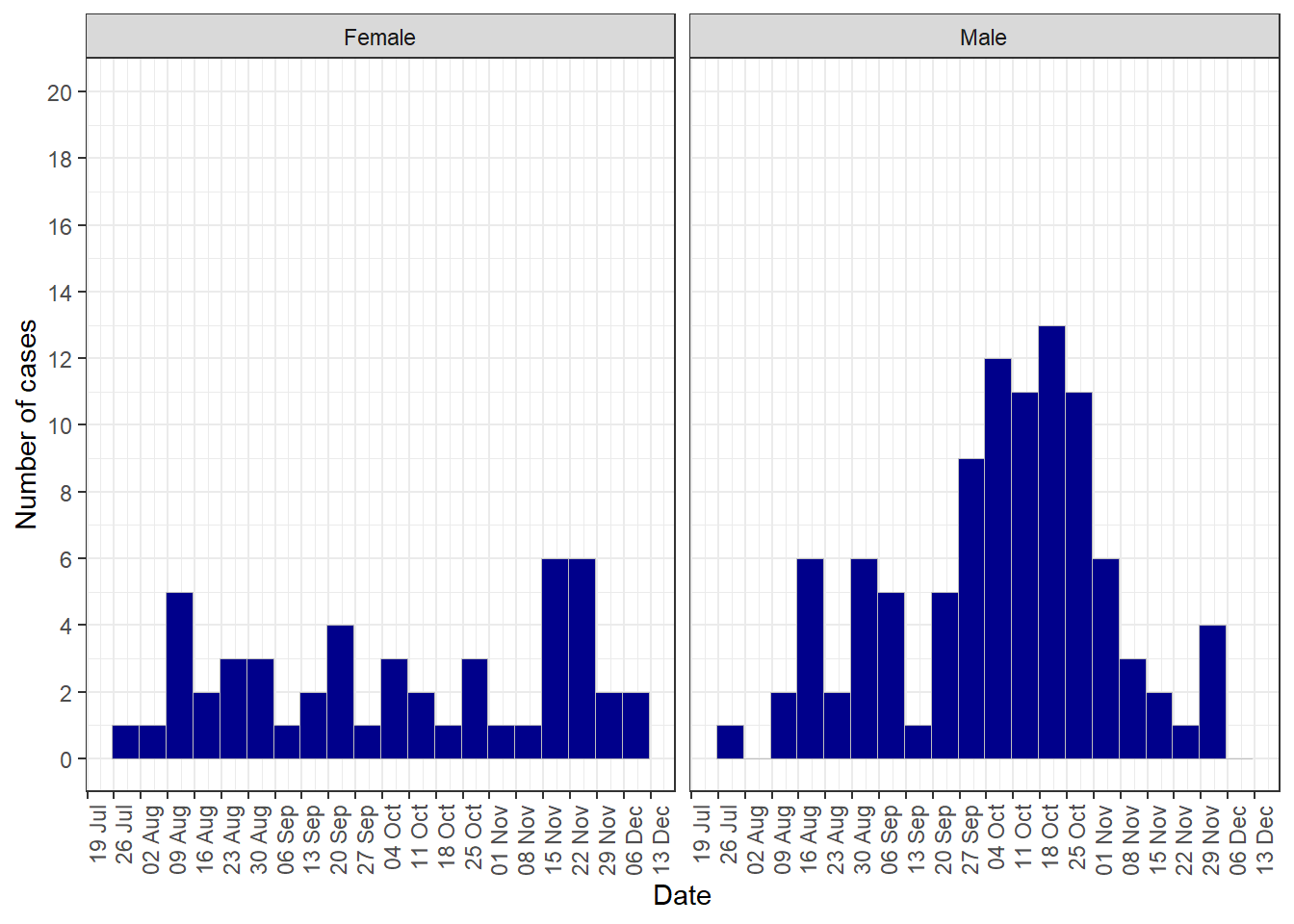Frequency histogram showing counts of incident cases of disease as a function of calendar date, 26 July to 13 December 2004, conditioned by sex.

Let’s say an event occurred on 31 October 2004. Mark this date on your epidemic curve using geom_vline:

ggplot(data = dat.df, aes(x = as.Date(odate))) +
theme_bw() +
geom_histogram(binwidth = 7, colour = "gray", fill = "dark blue", size = 0.1) +
scale_x_date(breaks = date_breaks("1 week"), labels = date_format("%d %b"),
name = "Date") +
scale_y_continuous(breaks = seq(from = 0, to = 20, by = 2), limits = c(0,20), name = "Number of cases") +
theme(axis.text.x = element_text(angle = 90, hjust = 1)) +
facet_grid( ~ sex) +
geom_vline(aes(xintercept = as.numeric(as.Date("31/10/2004", format = "%d/%m/%Y"))),
linetype = "dashed")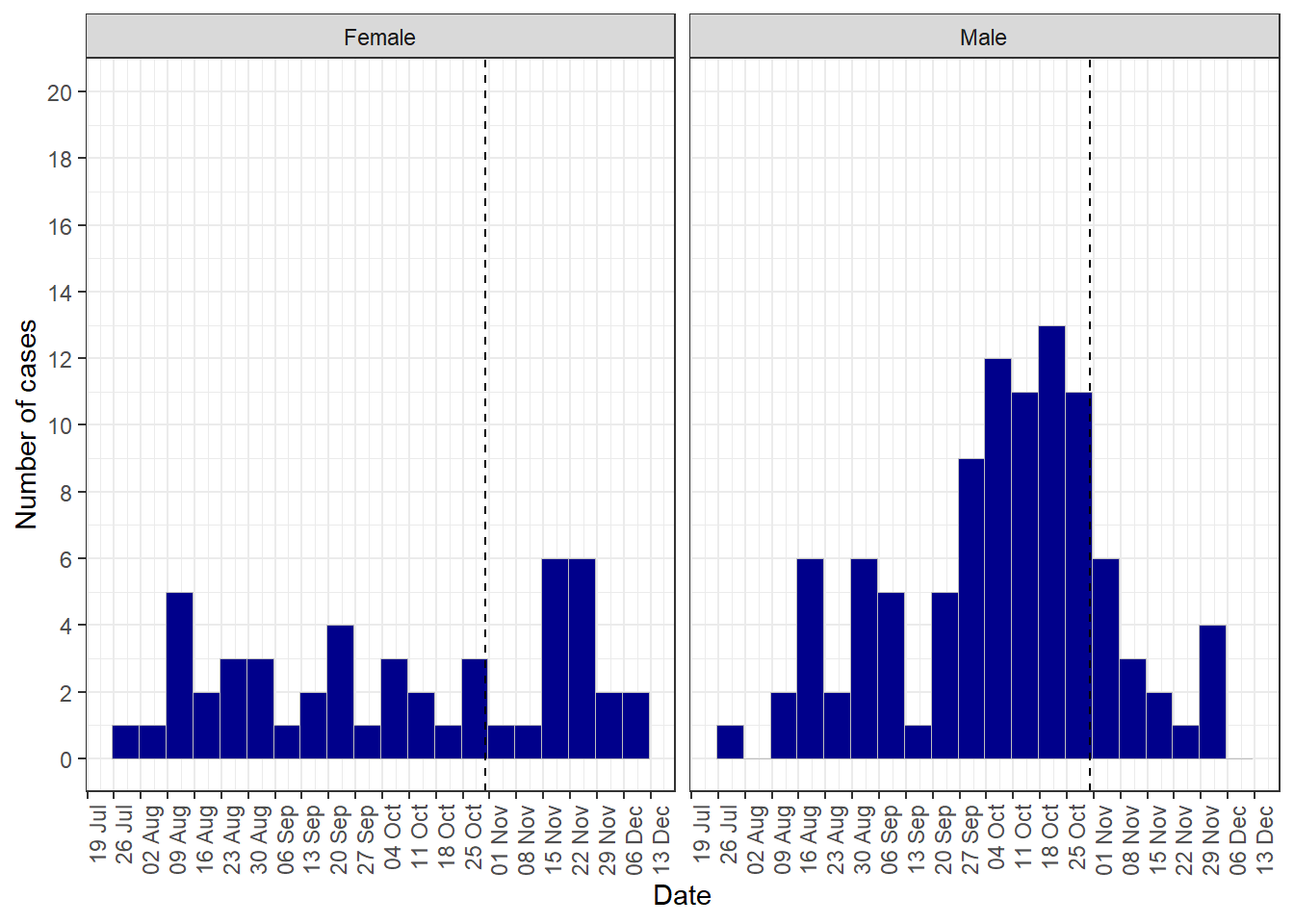Frequency histogram showing counts of incident cases of disease as a function of calendar date, 26 July to 13 December 2004, conditioned by sex. An event that occurred on 31 October 2004 is indicated by the vertical dashed line.

Plot the total number of disease events by day, coloured according to sex:

ggplot(data = dat.df, aes(x = as.Date(odate), group = sex, fill = sex)) +
theme_bw() +
geom_histogram(binwidth = 7, colour = "gray", size = 0.1) +
scale_x_date(breaks = date_breaks("1 week"), labels = date_format("%d %b"),
name = "Date") +
scale_y_continuous(breaks = seq(from = 0, to = 20, by = 2), limits = c(0,20), name = "Number of cases") +
theme(axis.text.x = element_text(angle = 90, hjust = 1)) +
geom_vline(aes(xintercept = as.numeric(as.Date("31/10/2004", format = "%d/%m/%Y"))),
linetype = "dashed") +
scale_fill_manual(values = c("#d46a6a", "#738ca6"), name = "Sex") +
theme(legend.position = c(0.90, 0.80))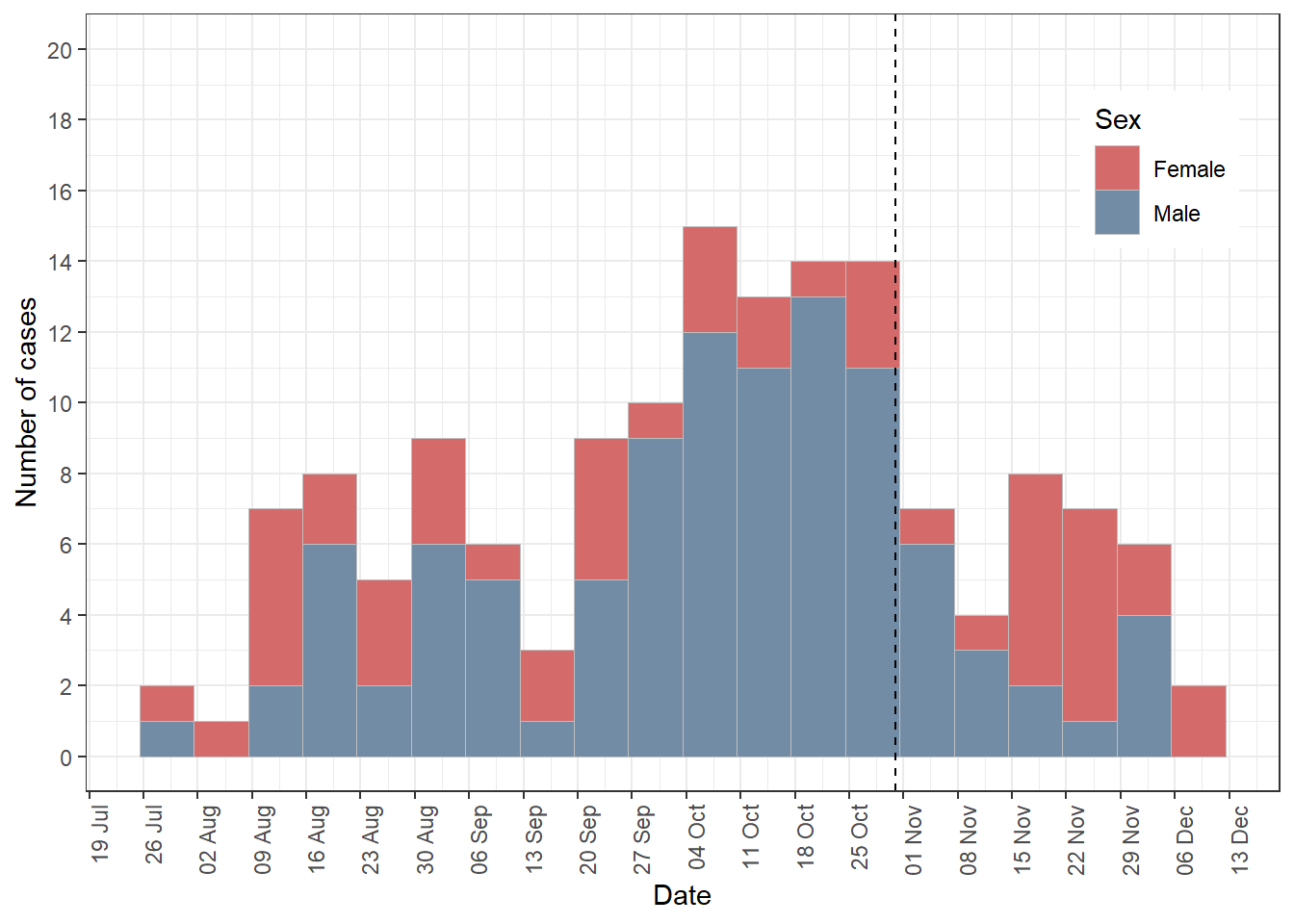Frequency histogram showing counts of incident cases of disease as a function of calendar date, 26 July to 13 December 2004, grouped by sex.

It can be difficult to appreciate differences in male and female disease counts as a function of date with the above plot format so dodge the data instead:

ggplot(data = dat.df, aes(x = as.Date(odate), group = sex, fill = sex)) +
theme_bw() +
geom_histogram(binwidth = 7, colour = "gray", size = 0.1, position = "dodge") +
scale_x_date(breaks = date_breaks("1 week"), labels = date_format("%d %b"),
name = "Date") +
scale_y_continuous(breaks = seq(from = 0, to = 20, by = 2), limits = c(0,20), name = "Number of cases") +
theme(axis.text.x = element_text(angle = 90, hjust = 1)) +
geom_vline(aes(xintercept = as.numeric(as.Date("31/10/2004", format = "%d/%m/%Y"))),
linetype = "dashed") +
scale_fill_manual(values = c("#d46a6a", "#738ca6"), name = "Sex") +
theme(legend.position = c(0.90, 0.80))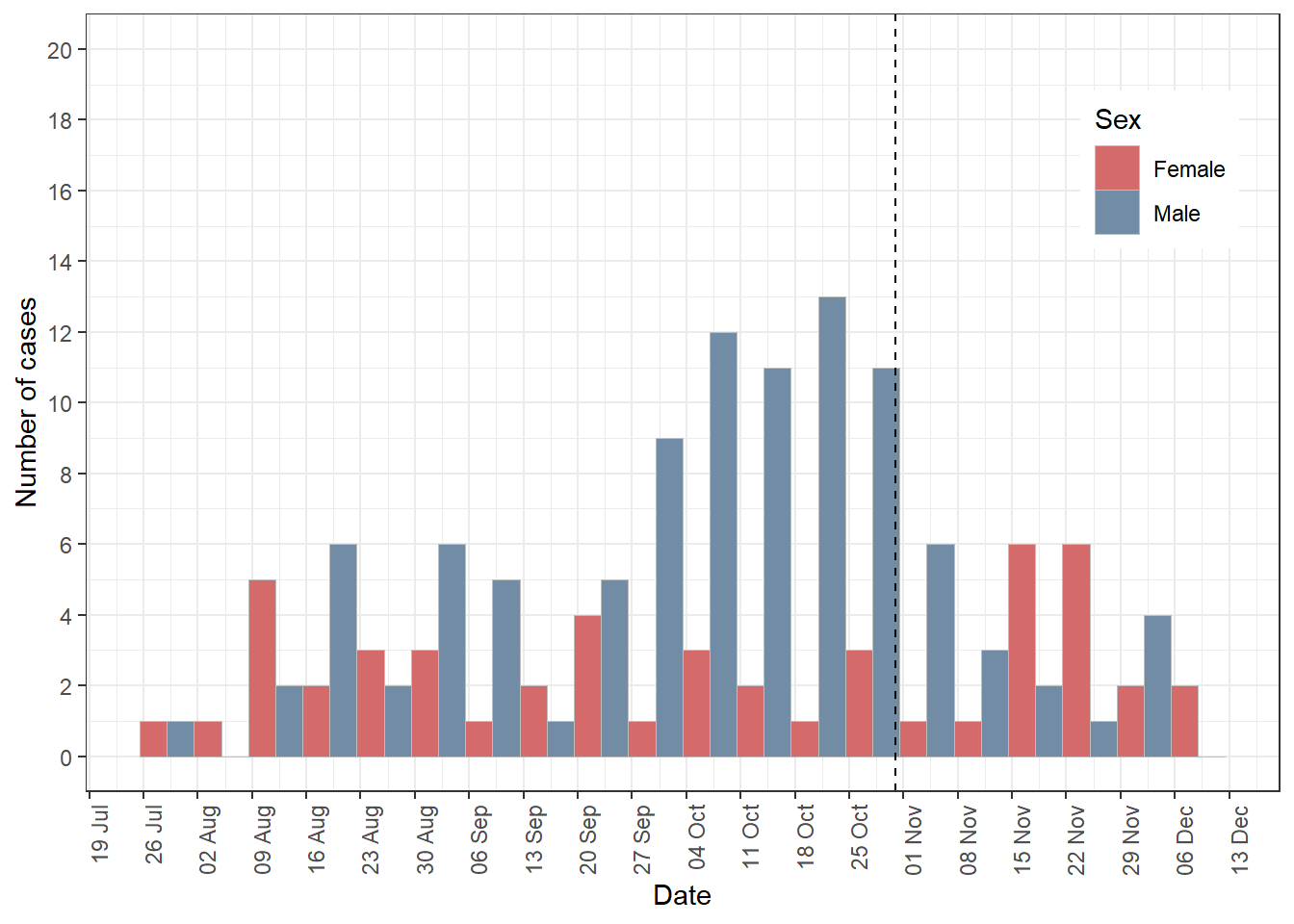Frequency histogram showing counts of incident cases of disease as a function of calendar date, 26 July to 13 December 2004, grouped by sex.

### Integers representing counts of cases on each date

We now provide code to deal with the situation where the data are presented with one row for every date during an outbreak and an integer representing the number of cases identified on each date.

Actual outbreak data will be used for this example. In the code below edate represents the event date (i.e., the date of case detection) and ncas represents the number of cases identified on each edate.

edate <- seq(from = as.Date("2020-02-24"), to = as.Date("2020-07-20"), by = 1)
ncas <- c(1,0,0,1,0,1,1,0,0,0,0,0,0,0,0,0,0,0,0,0,0,0,0,0,0,0,0,0,2,0,0,1,0,0,0,0,2,
0,0,1,0,1,1,2,3,2,5,10,15,5,7,17,37,31,34,42,46,73,58,67,57,54,104,77,52,
90,59,64,61,21,26,25,32,24,14,11,23,6,8,9,4,5,7,14,14,1,5,1,1,5,3,3,1,3,3,
7,5,10,11,21,14,16,15,13,13,8,5,16,7,9,19,13,5,6,6,5,5,10,9,2,2,5,8,10,6,
8,8,4,9,7,8,3,1,4,2,0,4,8,5,8,10,12,8,20,16,11,25,19)

dat.df <- data.frame(edate, ncas)
dat.df$edate <- as.Date(dat.df$edate, format = "%Y-%m-%d")
#>        edate ncas
#> 1 2020-02-24    1
#> 2 2020-02-25    0
#> 3 2020-02-26    0
#> 4 2020-02-27    1
#> 5 2020-02-28    0
#> 6 2020-02-29    1

Generate an epidemic curve. Note weight = ncas in the aesthetics argument for ggplot2:

ggplot() +
theme_bw() +
geom_histogram(dat.df, mapping = aes(x = edate, weight = ncas), binwidth = 1, fill = "#738ca6", colour = "grey", size = 0.1) +
scale_x_date(breaks = date_breaks("2 weeks"), labels = date_format("%b %Y"),
name = "Date") +
scale_y_continuous(limits = c(0,125), name = "Number of cases") +
theme(axis.text.x = element_text(angle = 90, hjust = 1))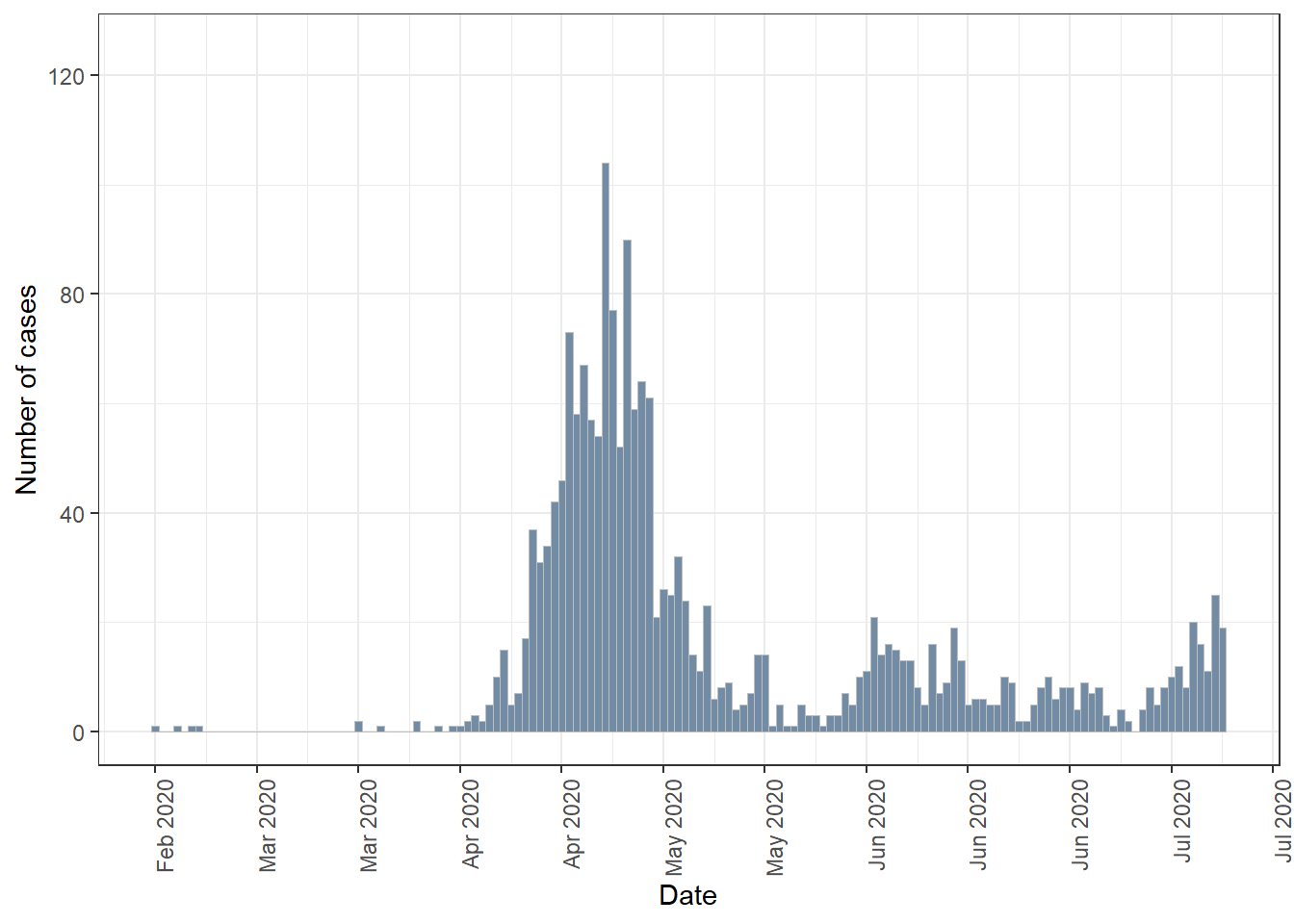Frequency histogram showing counts of incident cases of disease as a function of calendar date, 24 February 2020 to 20 July 2020.

This plot has features of a common point source epidemic for the period April 2020 to May 2020. After May 2020 the plot shows feature of a propagated epidemic pattern.

Add a line to the plot to show the cumulative number of cases detected as a function of calendar date. The coding here requires some thought. First question: What was the cumulative number of cases at the end of the follow-up period? Use the cumsum (cumulative sum) function in base R:

max(cumsum(dat.df$ncas)) #>  1834 At the end of the follow-up period the cumulative number of cases was 1834. What we need to do is to get our 0 to 1834 cumulative case numbers to ‘fit’ into the 0 to 125 vertical axis limits of the epidemic curve. A reasonable approach would be to: (1) divide cumulative case numbers by a number so that the maximum cumulative case number divided by our selected number roughly equals the maximum number of cases identified per day; for this example, 15 would be a good choice (1834 / 15 = 122); and (2) set sec.axis = sec_axis(~ . * 15) to multiply the values that appear on the primary vertical axis by 15 for the labels that appear on the secondary vertical axis:  ggplot() + theme_bw() + geom_histogram(data = dat.df, mapping = aes(x = edate, weight = ncas), binwidth = 1, fill = "#738ca6", colour = "grey", size = 0.1) + geom_line(data = dat.df, mapping = aes(x = edate, y = cumsum(ncas) / 15)) + scale_x_date(breaks = date_breaks("2 weeks"), labels = date_format("%b %Y"), name = "Date") + scale_y_continuous(limits = c(0,125), name = "Number of cases", sec.axis = sec_axis(~ . * 15, name = "Cumulative number of cases")) + guides(fill = "none") + theme(axis.text.x = element_text(angle = 90, hjust = 1))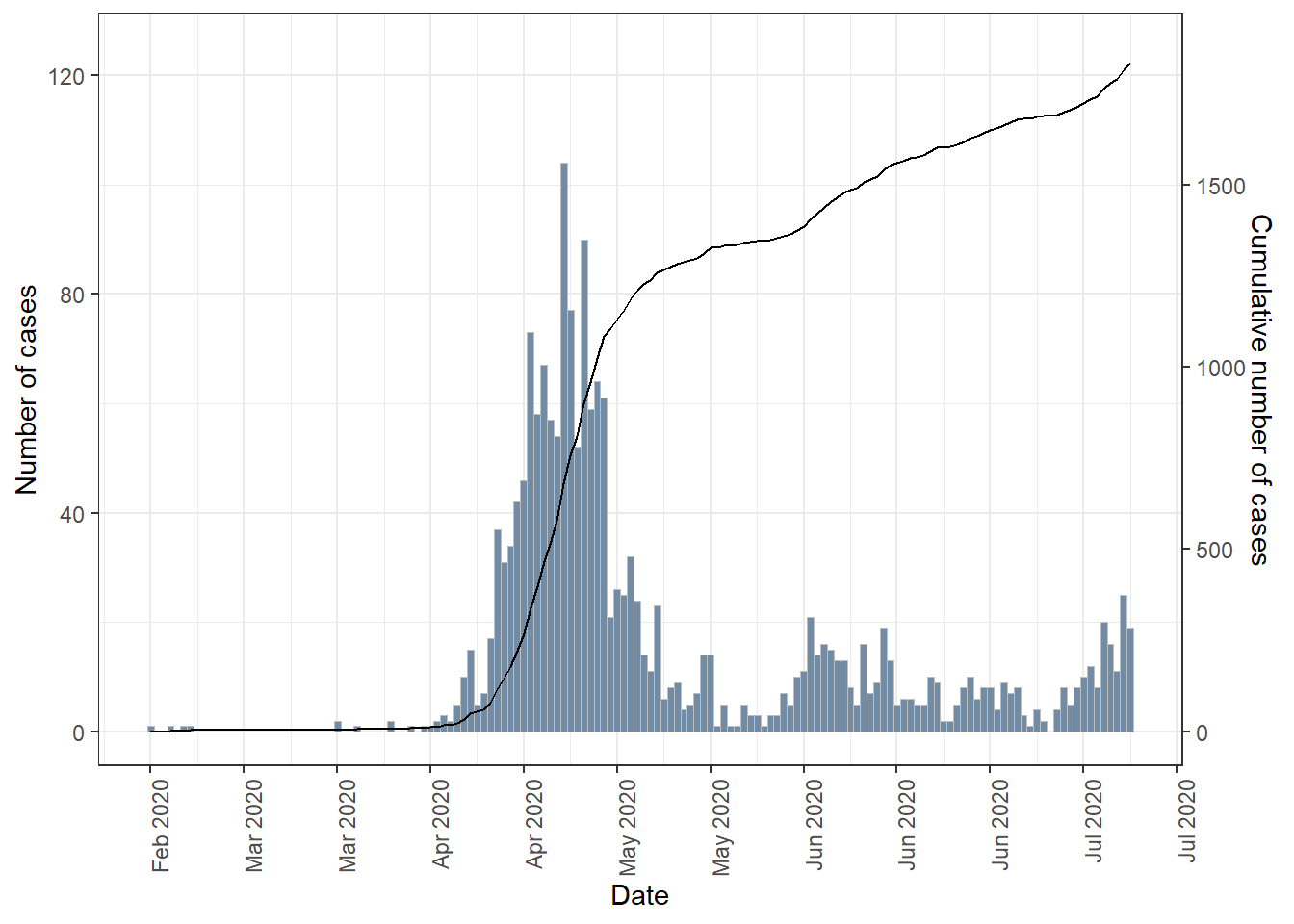Frequency histogram showing counts of incident cases of disease as a function of calendar date, 24 February 2020 to 20 July 2020. Superimposed on this plot is a line showing cumulative case numbers. Finally, we might want to superimpose a line representing the rolling average of case numbers. Calculate the 5-day rolling mean use the rollmean function in the contributed zoo package:  dat.df$rncas <- rollmean(x = dat.df$ncas, k = 5, fill = NA) ggplot() + theme_bw() + geom_histogram(data = dat.df, mapping = aes(x = edate, weight = ncas), binwidth = 1, fill = "#738ca6", colour = "grey", size = 0.1) + geom_line(data = dat.df, mapping = aes(x = edate, y = dat.df$rncas), colour = "red") +
scale_x_date(breaks = date_breaks("2 weeks"), labels = date_format("%b %Y"),
name = "Date") +
scale_y_continuous(limits = c(0,125), name = "Number of cases") +
guides(fill = "none") +
theme(axis.text.x = element_text(angle = 90, hjust = 1))
#> Warning: Use of dat.df$rncas is discouraged. Use rncas instead. #> Warning: Removed 4 row(s) containing missing values (geom_path).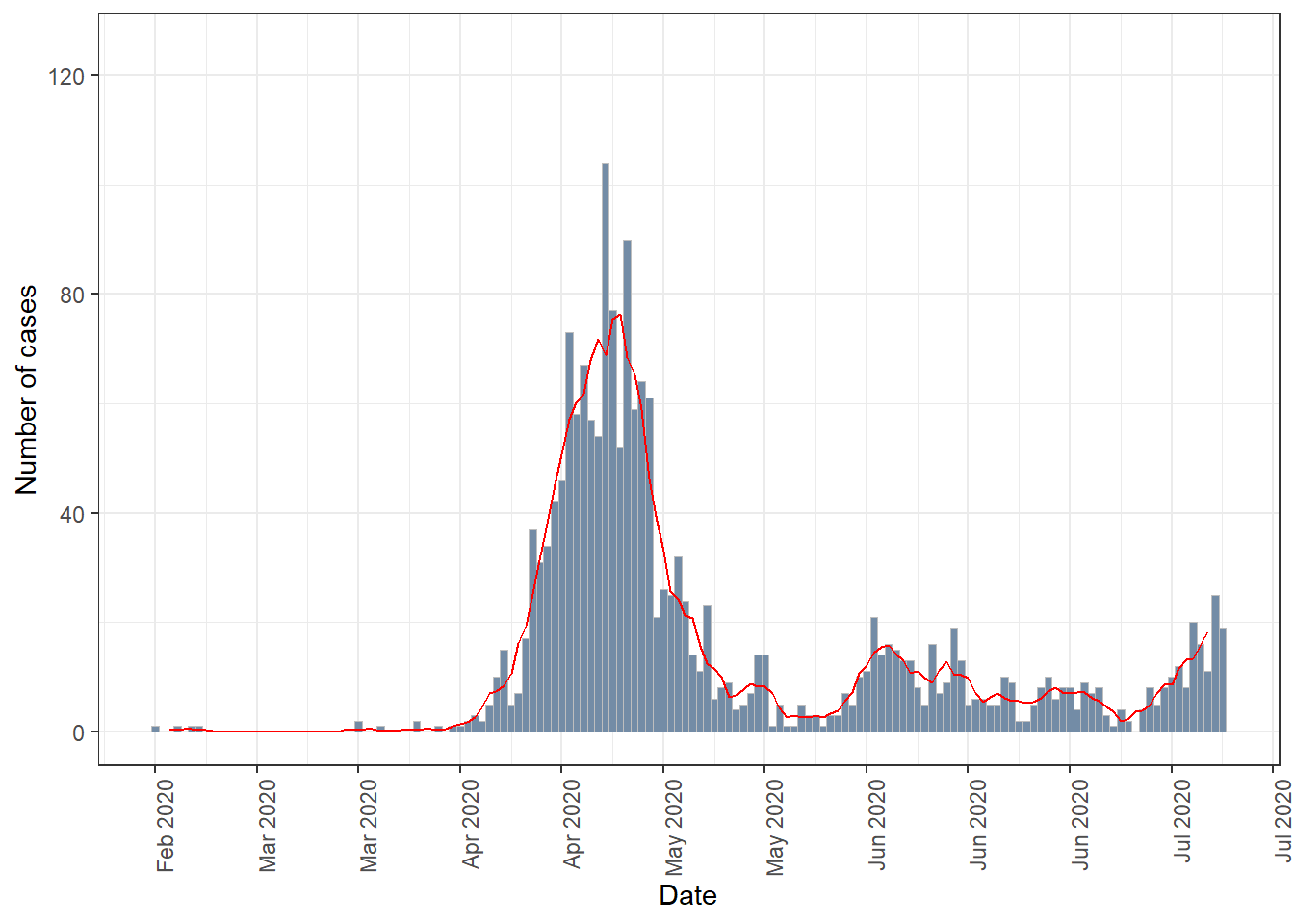Frequency histogram showing counts of incident cases of disease as a function of calendar date, 24 February 2020 to 20 July 2020. Superimposed on this plot is the 5-day rolling mean number of cases per day. ## Place Two types of maps are often used when describing patterns of disease by place: 1. Choropleth maps. Choropleth mapping involves producing a summary statistic of the outcome of interest (e.g. count of disease events, prevalence, incidence) for each component area within a study region. A map is created by ‘filling’ (i.e. colouring) each component area with colour, providing an indication of the magnitude of the variable of interest and how it varies geographically. 2. Point maps. Choropleth maps For illustration we make a choropleth map of sudden infant death syndrome (SIDS) babies in North Carolina counties for 1974 using the nc.sids data provided with the spData package. library(sf); library(spData); library(rgdal); library(plyr); library(RColorBrewer); library(spatstat) ncsids.sf <- st_read(dsn = system.file("shapes/sids.shp", package = "spData")) #> Reading layer sids' from data source #> C:\Program Files\R\R-4.2.1\library\spData\shapes\sids.shp' #> using driver ESRI Shapefile' #> Simple feature collection with 100 features and 22 fields #> Geometry type: MULTIPOLYGON #> Dimension: XY #> Bounding box: xmin: -84.32385 ymin: 33.88199 xmax: -75.45698 ymax: 36.58965 #> CRS: NA ncsids.sf <- ncsids.sf[,c("BIR74","SID74")] head(ncsids.sf) #> Simple feature collection with 6 features and 2 fields #> Geometry type: MULTIPOLYGON #> Dimension: XY #> Bounding box: xmin: -81.74107 ymin: 36.07282 xmax: -75.77316 ymax: 36.58965 #> CRS: NA #> BIR74 SID74 geometry #> 1 1091 1 MULTIPOLYGON (((-81.47276 3... #> 2 487 0 MULTIPOLYGON (((-81.23989 3... #> 3 3188 5 MULTIPOLYGON (((-80.45634 3... #> 4 508 1 MULTIPOLYGON (((-76.00897 3... #> 5 1421 9 MULTIPOLYGON (((-77.21767 3... #> 6 1452 7 MULTIPOLYGON (((-76.74506 3... The ncsids.sf simple features object lists for each county in the North Carolina USA the number SIDS deaths for 1974. Plot a choropleth map of the counties of the North Carolina showing SIDS counts for 1974: ggplot() + theme_bw() + geom_sf(data = ncsids.sf, aes(fill = SID74), colour = "dark grey") + scale_fill_gradientn(limits = c(0,60), colours = brewer.pal(n = 5, "Reds"), guide = "colourbar") + scale_x_continuous(name = "Longitude") + scale_y_continuous(name = "Latitude") + labs(fill = "SIDS 1974")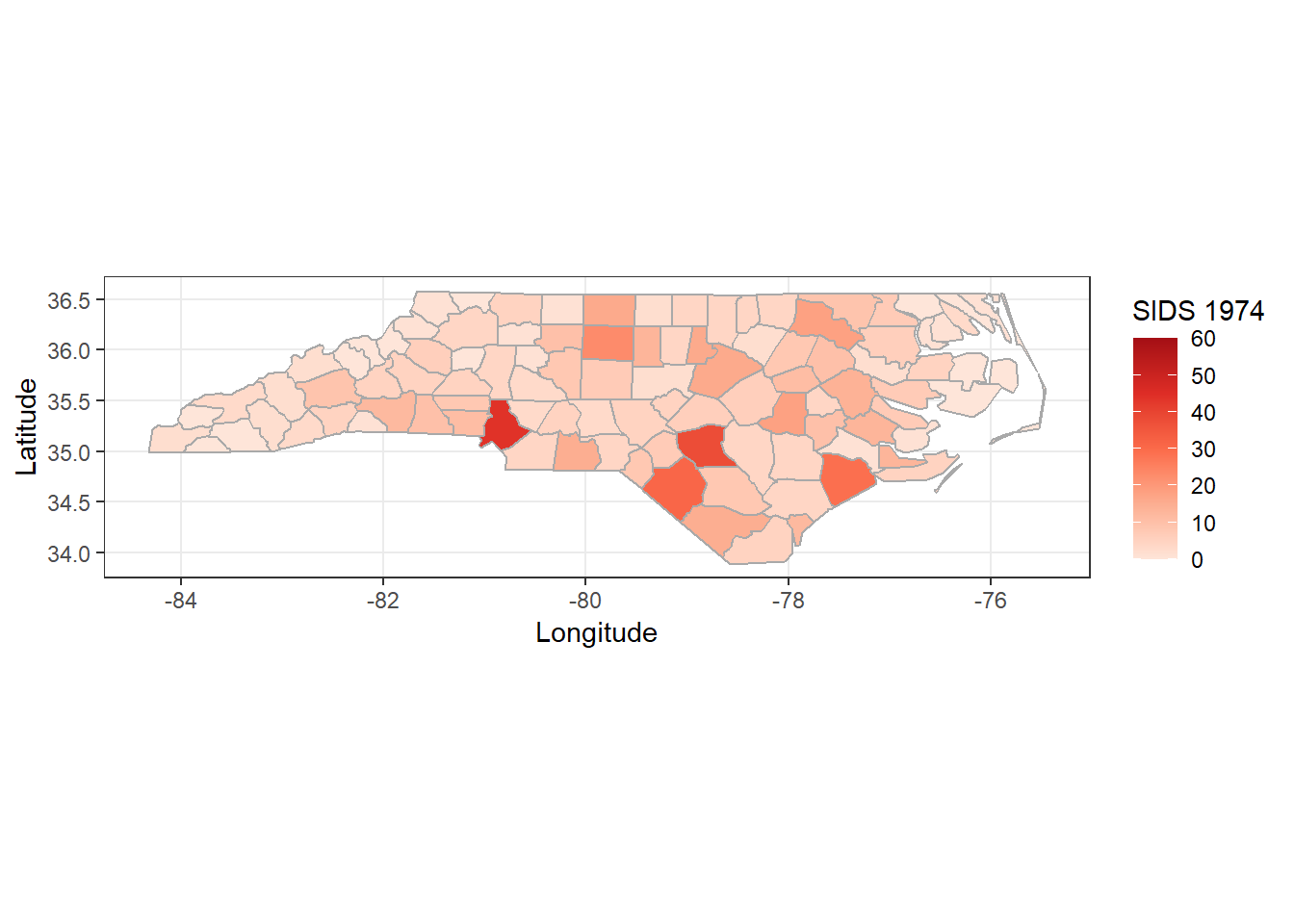Map of North Carolina, USA showing the number of sudden infant death syndrome cases, by county for 1974. Point maps For this example we will used the epi.incin data set included with epiR. Between 1972 and 1980 an industrial waste incinerator operated at a site about 2 kilometres southwest of the town of Coppull in Lancashire, England. Addressing community concerns that there were greater than expected numbers of laryngeal cancer cases in close proximity to the incinerator Diggle (1990) conducted a study investigating risks for laryngeal cancer, using recorded cases of lung cancer as controls. The study area is 20 km x 20 km in size and includes location of residence of patients diagnosed with each cancer type from 1974 to 1983. Load the epi.incin data set and create negative and positive labels for each point location. We don’t have a boundary map for these data so we’ll use spatstat to create a convex hull around the points and dilate the convex hull by 1000 metres as a proxy boundary. The point locations in this data are projected using the British National Grid coordinate reference system (EPSG code 27700). Create an observation window for the data as coppull.ow and a ppp object for plotting: data(epi.incin); incin.df <- epi.incin incin.df$status <- factor(incin.df$status, levels = c(0,1), labels = c("Neg", "Pos")) names(incin.df) <- "Status" incin.sf <- st_as_sf(incin.df, coords = c("xcoord","ycoord"), remove = FALSE) st_crs(incin.sf) <- 27700 coppull.ow <- convexhull.xy(x = incin.df[,1], y = incin.df[,2]) coppull.ow <- dilation(coppull.ow, r = 1000) Create a simple features polygon object from coppull.ow. First we convert coppull.ow to a SpatialPolygonsDataFrame object: coords <- matrix(c(coppull.ow$bdry[]$x, coppull.ow$bdry[]\$y), ncol = 2, byrow = FALSE)
pol <- Polygon(coords, hole = FALSE)
pol <- Polygons(list(pol),1)
pol <- SpatialPolygons(list(pol))
coppull.spdf <- SpatialPolygonsDataFrame(Sr = pol, data = data.frame(id = 1), match.ID = TRUE)

Convert the SpatialPolygonsDataFrame to an sf object and set the coordinate reference system:

coppull.sf <- as(coppull.spdf, "sf")
st_crs(coppull.sf) <- 27700

The mformat function is used to plot the axis labels in kilometres (instead of metres):

mformat <- function(){
function(x) format(x / 1000, digits = 2)
}
ggplot() +
theme_bw() +
geom_sf(data = incin.sf, aes(colour = Status, shape = Status)) +
geom_sf(data = coppull.sf, fill = "transparent", colour = "black") +
coord_sf(datum = st_crs(coppull.sf)) +
scale_colour_manual(values = c("grey","red")) +
scale_shape_manual(values = c(1,16)) +
scale_x_continuous(name = "Easting (km)", labels = mformat()) +
scale_y_continuous(name = "Northing (km)", labels = mformat()) +
theme(legend.position = c(0.10, 0.15))`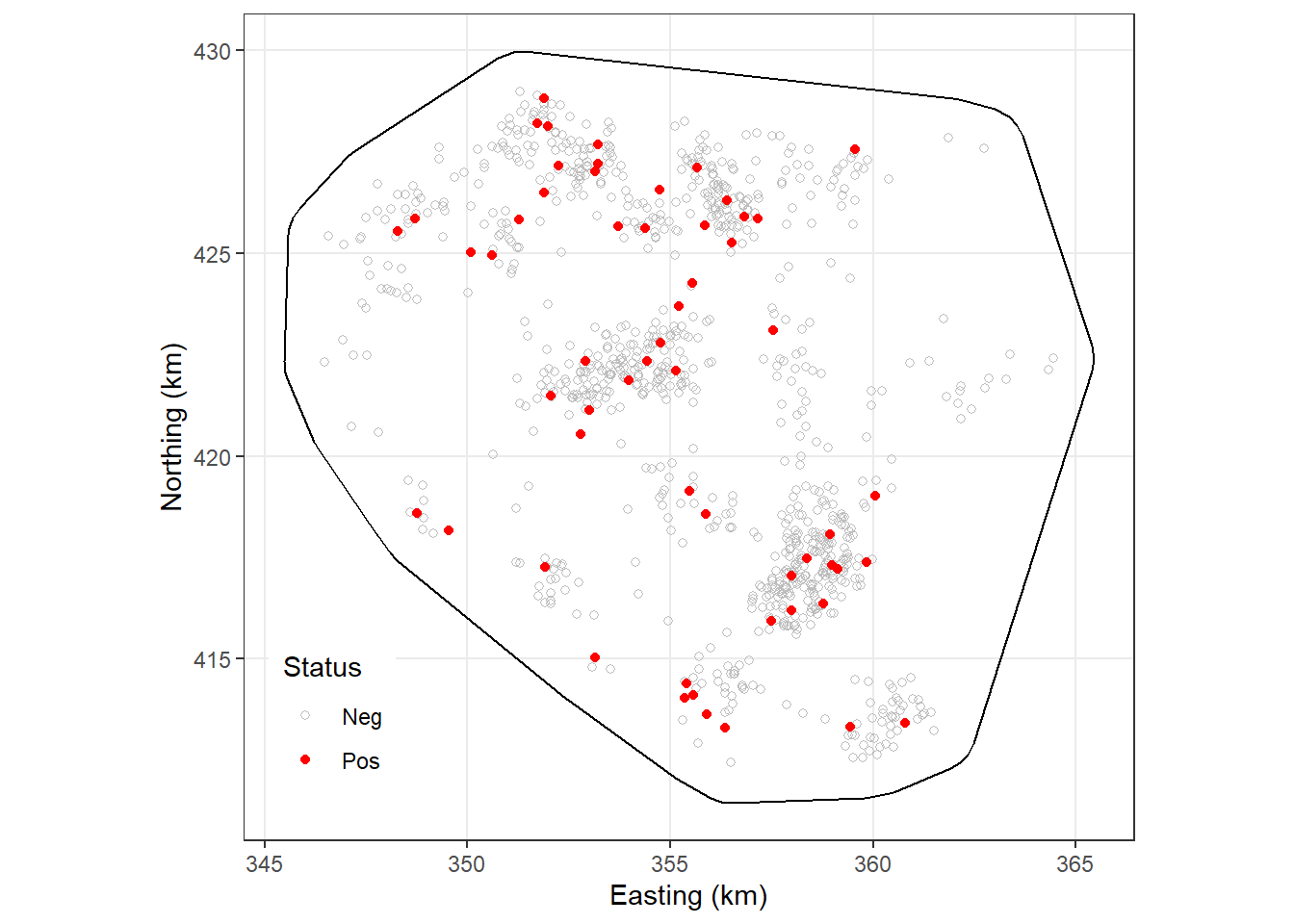Point map showing the place of residence of individuals diagnosed with laryngeal cancer (Pos) and lung cancer (Neg), Copull Lancashire, UK, 1972 to 1980.